• 正文
• 相关推荐
• 电子产业图谱

# 自制仿生太阳追踪器—向日葵舞者

2022/10/22346扫码加入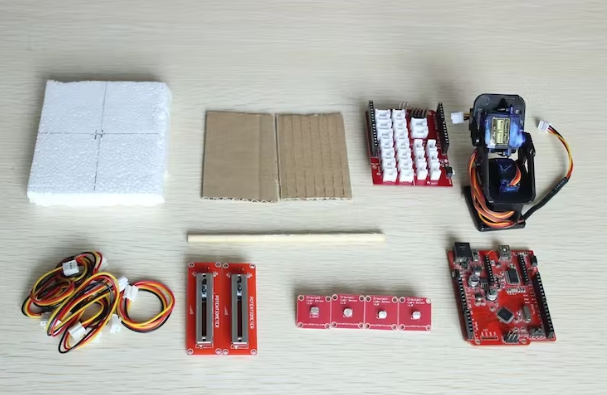• 纸板x 2
• 泡沫聚苯乙烯板× 1
• 把x 1
• 3引脚斜尾电缆x 6
• 线性电位器x 2
• 光传感器x 4
• Crowduino ATMega 328 V1.1 x 1
• Crowtail- Base Shield Arduino x 1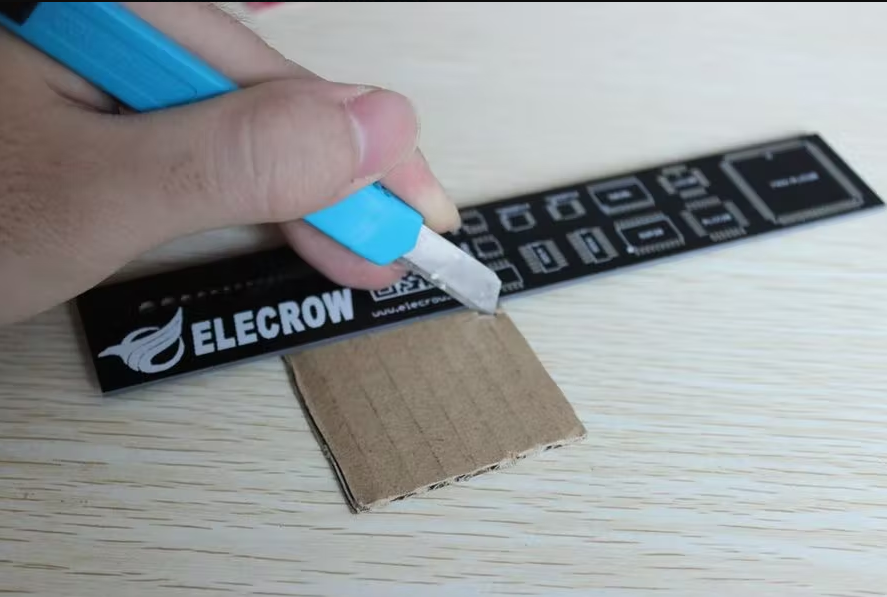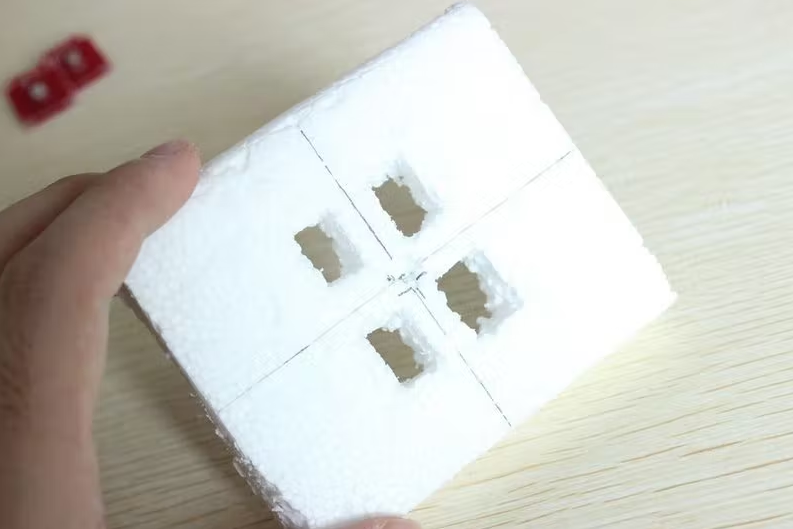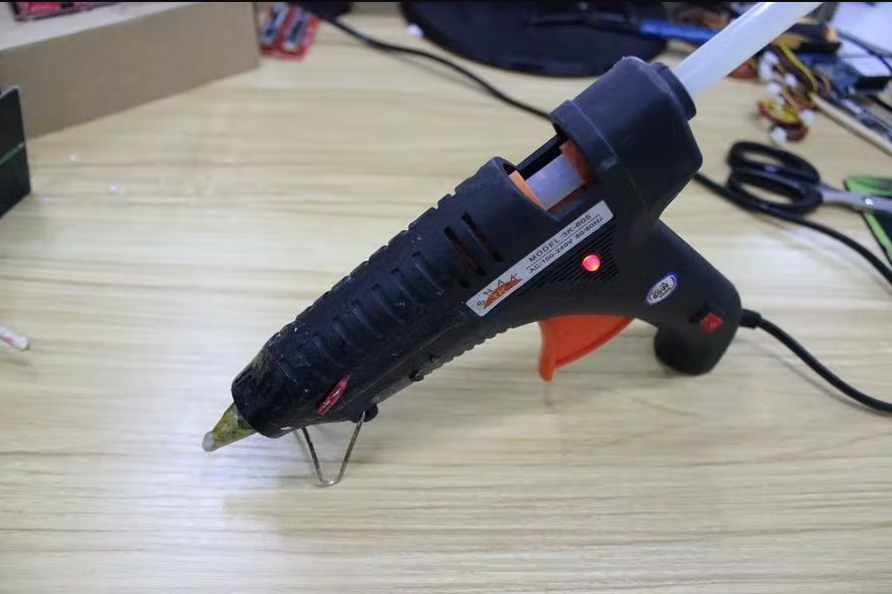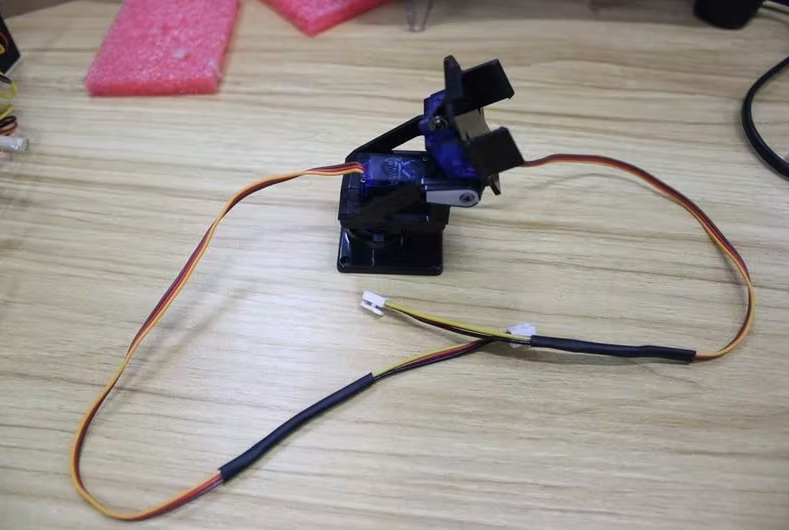pan-tilt是一个配有9G伺服的组装装置。可控制垂直和水平180度旋转。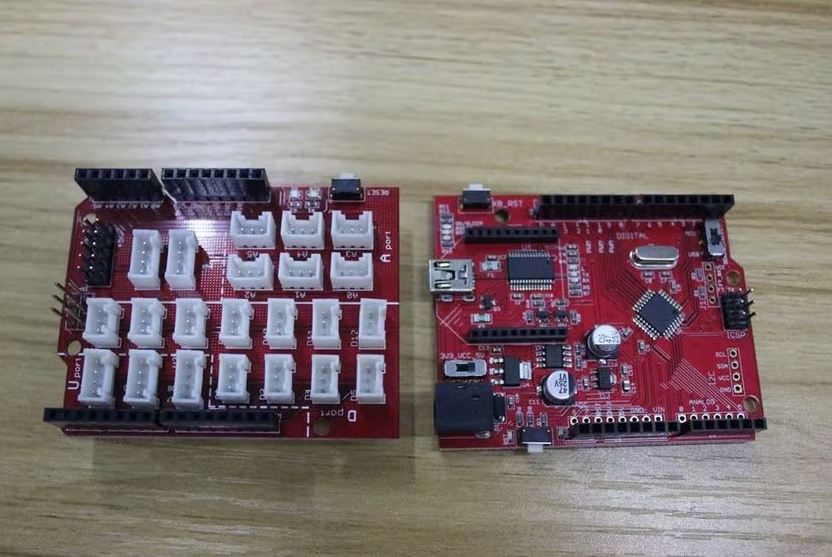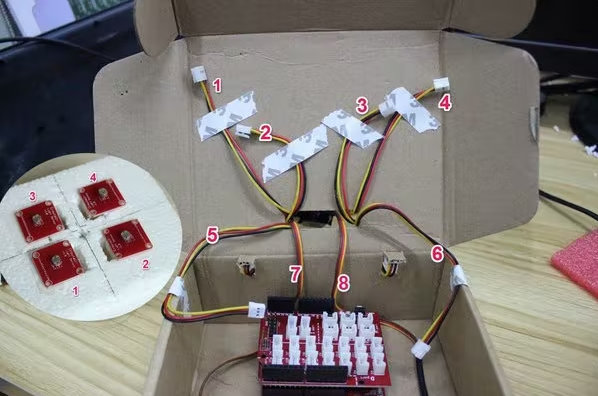1 - 4电缆:

• 电缆“1”到A0
• 电缆“2”到A1
• 电缆“4”到A2
• 电缆“3”到A3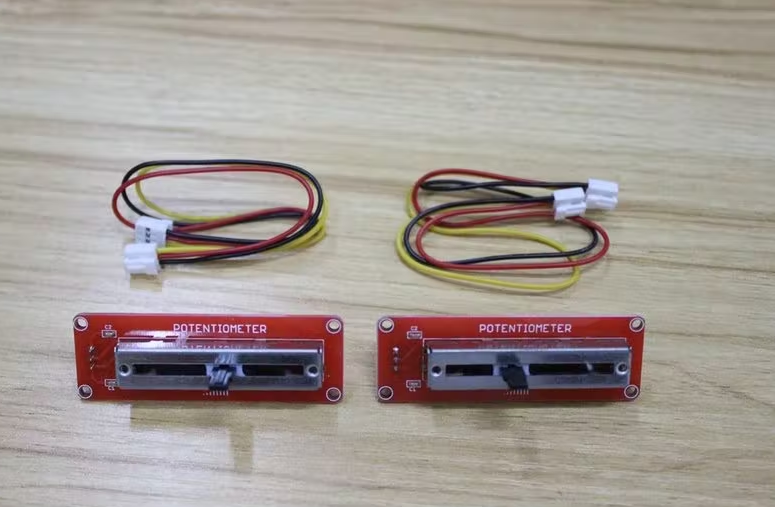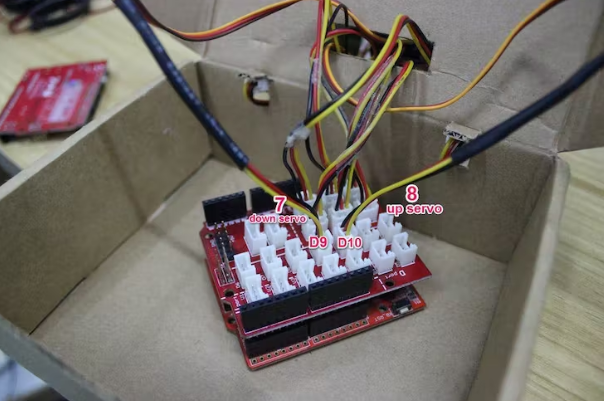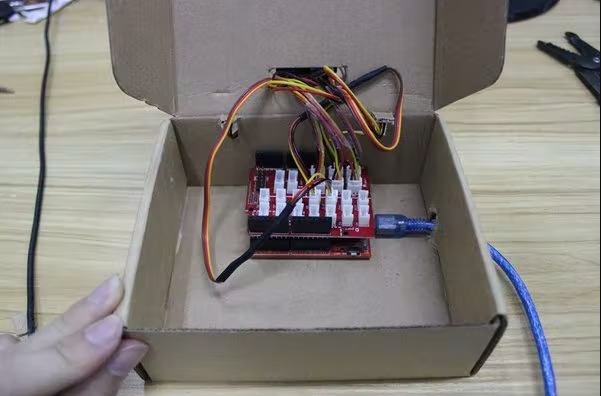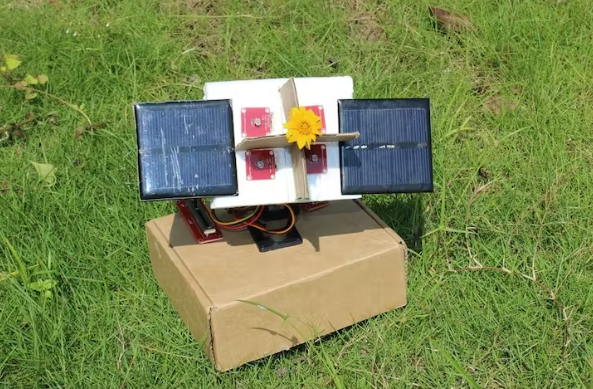arduino向日葵代码：

#include <Servo.h> // include Servo library

Servo horizontal; // horizontal servo
int servoh = 90; // stand horizontal servo

Servo vertical; // vertical servo
int servov = 90; // stand vertical servo

// LDR pin connections
// name = analogpin;
int ldrrd =0;
int ldrld =1;
int ldrlt = 2;
int ldrrt = 3;

void setup()
{
Serial.begin(9600);
// servo connections
// name.attacht(pin);
horizontal.attach(9);
vertical.attach(10);
}

void loop()
{
int lt = analogRead(ldrlt); // top left
int rt = analogRead(ldrrt); // top right
int ld = analogRead(ldrld); // down left
int rd = analogRead(ldrrd); // down rigt

int avt = (lt + rt) / 2; // average value top
int avd = (ld + rd) / 2; // average value down
int avl = (lt + ld) / 2; // average value left
int avr = (rt + rd) / 2; // average value right

int dvert = avt - avd; // check the diffirence of up and down
int dhoriz = avl - avr;// check the diffirence og left and rigt

if (-1*tol > dvert || dvert > tol) // check if the diffirence is in the tolerance else change vertical angle
{
if (avt > avd)
{
servov = ++servov;
if (servov > 180)
{
servov = 180;
}
}
else if (avt < avd)
{
servov= --servov;
if (servov < 0)
{
servov = 0;
}
}
vertical.write(servov);
}

if (-1*tol > dhoriz || dhoriz > tol) // check if the diffirence is in the tolerance else change horizontal angle
{
if (avl > avr)
{
servoh = --servoh;
if (servoh < 0)
{
servoh = 0;
}
}
else if (avl < avr)
{
servoh = ++servoh;
if (servoh > 180)
{
servoh = 180;
}
}
else if (avl == avr)
{
// nothing
}
horizontal.write(servoh);
}
delay(dtime);